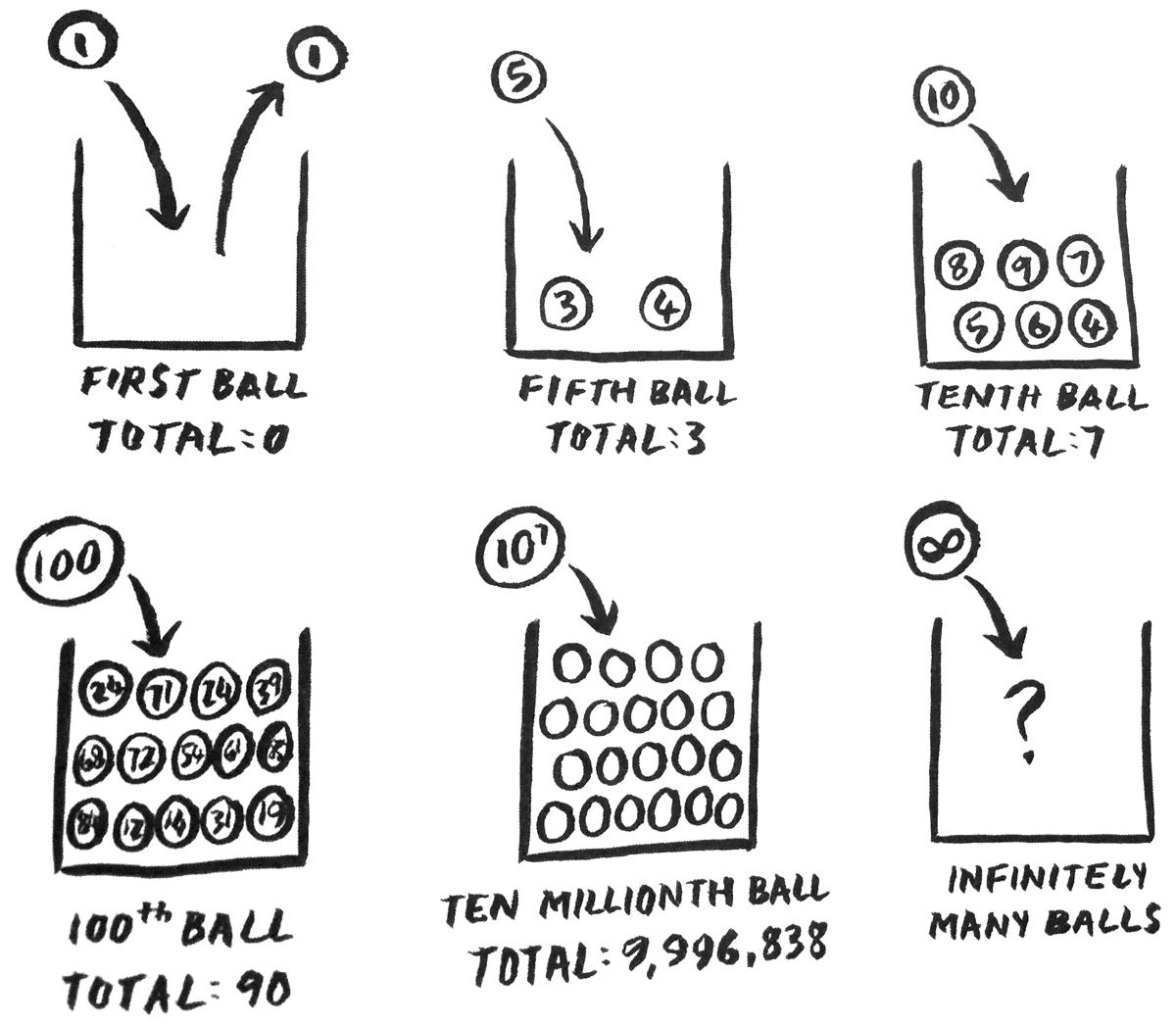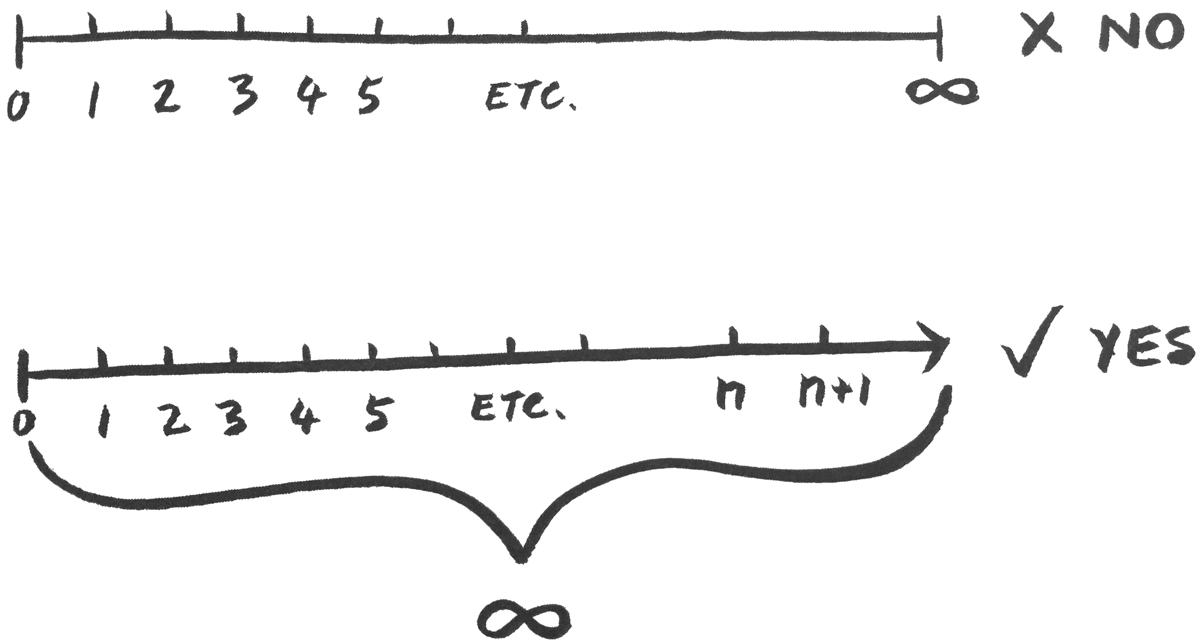/ / 1 am Wed, Jan 7 2015

# How to have mind-boggling fun with infinity# How to have mind-boggling fun with infinity

The human brain is happy working with nice finite numbers. As soon as you start working with infinity, things start happening that are completely counter-intuitive.

Infinity is like a loose thread on the coat of mathematics. If you start pulling on it, not only is it longer than expected, but everything else starts to unravel and suddenly you’re standing there, mathematically naked, wishing you could push that thread back in and continue happily ignoring it.

But not us. We’re going to grab that thread. We’re going to go to infinity and, indeed, beyond.

Making and doing things infinitely can get a bit tricky, but we’ll give it a go. Find yourself a box and a lot of balls and number the balls, starting 1, 2, 3, 4 . . . You get the picture. The game is to put the balls in the box one at a time, starting with ball number 1, but, whenever you put in a square-number ball, you take out its square root and put it away in a drawer or somewhere safe. This means that the first move is a bit odd, because when you put in ball 1, 1 is its own square root, so you take it straight back out. Then you can put in balls 2 and 3. When ball 4 goes in, you take ball 2 back out and put it in the drawer. Then balls 5 through to 8 go in, before adding ball 9 and taking out ball 3. The question is: if you simply keep doing this, which balls will end up in the box? How many are safe in the drawer?The surprise is that the box ends up completely empty. Now, this doesn’t make sense, because, as you’re going through the process, the number of balls in the box always goes up. At each move, you either add a ball, or you add one and take one away at the same time: the number of balls either increases or stays the same. But all the balls end up in the drawer, not the box. So when are all the balls dumped back out of the box?

We know all the balls end up in the drawer because every number can be squared. For each ball you put into the box, there is another ball out there which is the square of its number, and that square-number ball has to be added sooner or later. So, every ball you put in will eventually be taken out, in theory leaving the box empty. There is no number so big that it cannot be squared. But yet, at every step, there are always more balls in the box than in the drawer.

This paradox – that there are always more balls in the box, but yet they end up in the drawer – is caused by our expectation that infinity behaves like a really, really big number. But infinity is not a number. Infinity is nowhere to be found on the number line. People seem to have the idea that if you keep counting up along the number line past bigger and bigger numbers, in the end, the numbers just give up. They can’t be bothered to go on, and there’ll be an infinity sign (∞) there to mark the end of the number line. This is not the case. There is always a bigger number. Infinity is not safely contained at the end of the number line. Infinity is not a big number.

Infinity is actually a measure of how many numbers there are. The number line never stops, so we say it is infinitely long. Just as the number "five" is the size of any set of five things, infinity is the size of a never-ending set of things. Imagine the set of whole numbers; that’s how big infinity is. That’s when the balls get dumped out of the box: when you go from moving up the never-ending number line to looking at the whole number line at once, when you go from thinking about a very big number to thinking about all the numbers.There are infinitely many whole numbers, and every single one of them is the square root of a whole number. Instead of imagining putting the balls into the box and the drawer, you could imagine doing it all in one step. All the balls that are square roots go straight into the drawer, and all the balls that are not go in the box. Not a single ball would be put in the box.

Our big problem is that infinity doesn’t make any sense as far as our intuition is concerned, and there are no footholds. Maybe our brain doesn’t like dealing with higher dimensions, but at least we’re OK dealing with lower dimensions. The human brain is happy working with nice finite numbers. As soon as you start working with infinity, things start happening that are completely counter-intuitive. Finite numbers are not a preparation for infinity in the same way that 3D shapes readied us for 4D shapes. If anything, our affinity with the finite gives us a false sense of security when we encounter the infinite. We have to let go of all intuition; otherwise, it will lead us astray. Mathematical logic can be our only guide in a world that no longer has any link to our physical reality.

Doing math without intuition is like taking a submarine below the surface of an ocean. Back in the math jungle, our intuition allowed us to look around and make sense of our surroundings. Back then, enough of the mats directly linked to the world around us. Descending into the deep, however, means we can no longer see where we are going. We have left our physical universe and plunged into a world of pure abstract thought. On a submarine, you have to rely solely on the readouts of the instruments, and, likewise, we now have only mathematical results to guide us. But if we are fastidious and check every step, and trust the conclusions we deduce from what our mathematical instruments are telling us, all will be well

Image: Shutterstock